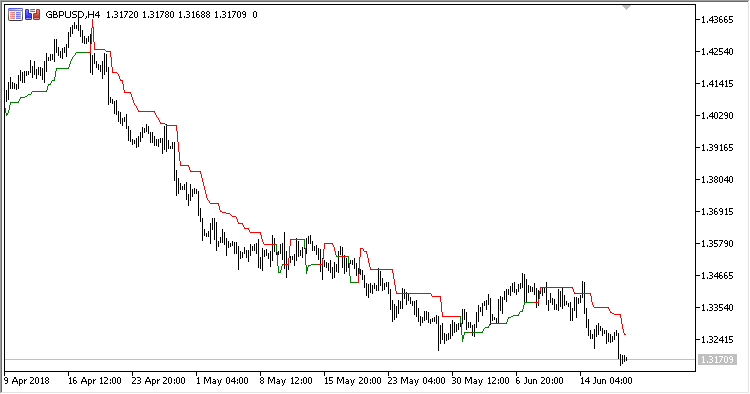# LeManStop – indicator for MetaTrader 5

• A+
category：MT5 INDICATORS

Trend-following indicator LeManStop is a representative of system LeMan and displays Stop Loss levels for the existing positions in the chart. It draws two Stop Loss lines for Long and Short positions, displayed in different colors.

It has six configurable parameters:

• Period - calculation period;
• Coefficient - coefficient of stop line deviation from the price;
• Fast MA period - fast moving average calculation period;
• Fast MA method - fast moving average calculation method;
• Slow MA period - slow moving average calculation period;
• Slow MA method - slow moving average calculation method.

Calculations:

If dMA > 0:

`LeMan Stop = PrevOpen - Coeff * SMA(HO, Period)`

If dMA < 0:

`LeMan Stop = PrevOpen + Coeff * SMA(OL, Period)`

where:

```HO = High - Open
OL = Open - Low
dMA = FastMA - SlowMA
FastMA - MA(Close, Fast MA period, Fast MA method)
SlowMA - MA(Close, Slow MA period, Slow MA method)
```